# Pyramid in Math: Definition & Practice Problems

Lesson Transcript
Instructor
Jennifer Beddoe

Jennifer has an MS in Chemistry and a BS in Biological Sciences.

Expert Contributor
Laura Pennington

Laura received her Master's degree in Pure Mathematics from Michigan State University, and her Bachelor's degree in Mathematics from Grand Valley State University. She has 20 years of experience teaching collegiate mathematics at various institutions.

It's not uncommon for one's first thought of a pyramid to be on the pyramids in Egypt, but pyramids actually are shapes that exist in multiple applications and different styles. Learn about the mathematical definition of a pyramid, the most common types, and practice using the formulas used to measure pyramids. Updated: 10/13/2021

## Types of Pyramids

A pyramid is a 3-dimensional shape whose base is a polygon. Each corner of a polygon is attached to a singular apex, which gives the pyramid its distinctive shape. Each base edge and the apex form a triangle.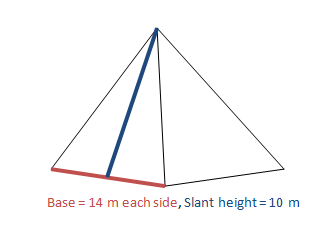There are many types of pyramids. Most often, they are named after the type of base they have. Let's look at some common types of pyramids below.

Triangular pyramid has a triangle as its base: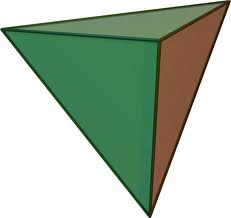Square pyramid has a square as its base: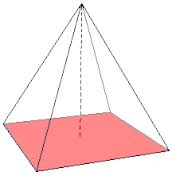Pentagonal pyramid has a pentagon as its base: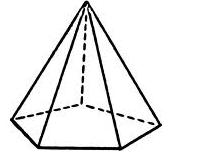This list could go on and on (hexagonal pyramid, heptagonal pyramid, etc). There are also a few special names for pyramids that you should know.

Right pyramid - the apex of the pyramid is directly above the center of its base: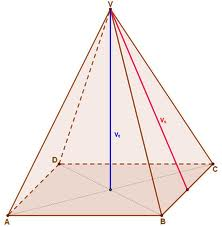Oblique pyramid - the apex of the pyramid is not directly above the center of its base: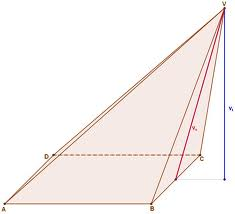Regular pyramid - the base of this pyramid is a regular polygon: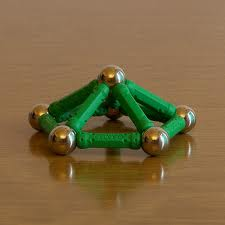Irregular pyramid - this type of pyramid has an irregular polygon as its base: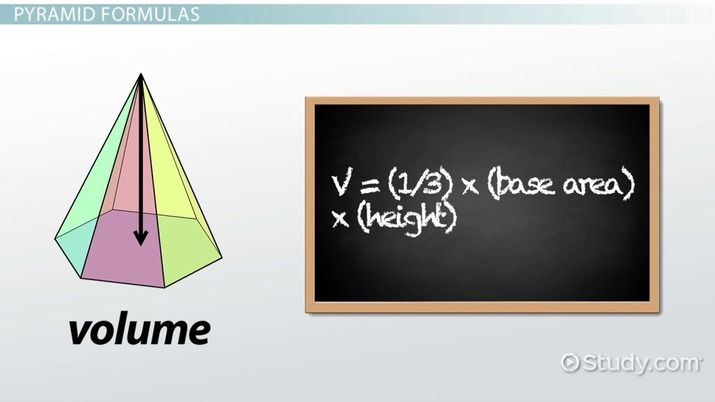An error occurred trying to load this video.

Try refreshing the page, or contact customer support.

Coming up next: Supplementary Angle: Definition & Theorem

### You're on a roll. Keep up the good work!

Replay
Your next lesson will play in 10 seconds
• 0:05 Types of Pyramids
• 1:09 Pyramid Formulas
• 2:36 Example Problems
• 4:19 Lesson Summary
Save Save

Want to watch this again later?

Timeline
Autoplay
Autoplay
Speed Speed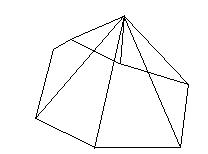## Pyramid Formulas

There are formulas that can be used to find both the surface area and the volume of a pyramid. The surface area of a pyramid is the total area of all the surfaces that the pyramid has. To that end, the formula for finding the surface area when all of the side faces are the same is:

SA = (base area) + (1/2) * (perimeter) * (slant height)

The base area is the area of the base and can be determined based on what figure the base is. For example, if the base of the pyramid is a square, the formula for finding the area is:

A = s^2

The perimeter is the distance around the base of the pyramid. The slant height is the diagonal height from the center of one of the base edges to the apex.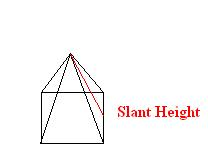If the pyramid has side faces that differ from each other (like in the case of an irregular pyramid), then the surface area equation is:

SA = (base height) + (lateral area)

In this case, you must take each side of the pyramid separately (including the base), find the areas, and then just add them together.

The volume of a pyramid can be found using this formula:

V = (1/3) * (base area) * (height)

The base area is, again, just the area of the base of the pyramid. However, in this case, the height is the length of a line from the apex that makes a right angle with the base.

## Example Problems

Let's take a look at some examples.

#### Example 1

A square pyramid has a height of 9 meters. If the side of the base measures 4 meters, what is the volume of the pyramid?

Since the base is a square, area of the base = 4 * 4 = 16 m^2.

Volume of the pyramid = (1/3) * (base area) * (height)

V = (1/3) * (16) * (9) = 48 m^3

#### Example 2

What is the surface area of the pyramid discussed in the first example?

In order to find the surface area, we must first find the slant height of the pyramid. Since we know the height and the base length, we can use the Pythagorean Theorem to find the slant length.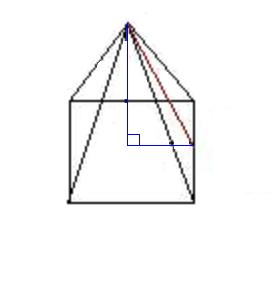You can see that the blue and red lines create a right triangle. The length of the long leg of the triangle is 9 meters, or the height of the triangle. The length of the short leg of the triangle is 2 meters because it is half the length of the base of the triangle (4 m). The red line is the hypotenuse of the triangle and can be calculated using the formula:

a^2 + b^2 = c^2

2^2 + 9^2 = c^2

81 + 4 = c^2

85 = c^2

c = 9.2 m

Now that you know the slant height, you can solve for the surface area of this triangle.

To unlock this lesson you must be a Study.com Member.

## Fill in the Blank Practice With Pyramids Activity

### Directions:

• Fill in the correct words or numbers in the story in the missing blanks.

### Story:

You are in Egypt, and you are on a group tour of the pyramids there. You come upon the first pyramid, and it is quite a sight due to its enormous size. The tour guide says the following:

• This is one of the larger pyramids here in Egypt. When it was first built, it was the tallest of all of the pyramids with a height of 50 meters, though taller and bigger pyramids have been built since then. The floor of this pyramid is a square with side lengths of 60 meters each, making it a _____ pyramid, and giving it a floor area of 3600 square meters and a floor perimeter of 240 square meters. The top tip of the pyramid lies directly above the center of the square floor, making it a _____ pyramid. People are still baffled today at how they Egyptians were able to build such magnificent structures. This pyramid, in particular, has a surface area (including the floor) of 9600 square meters, so it took that much building material to create! To do this, builders had to have ways of traveling up the sides of the pyramid to the top tip. This was done starting at the midpoint of one of the sides of the square floor up the side of the pyramid to the top tip of the pyramid, a distance of _____ meters.

You and the group are discussing different theories on how the Ancient Egyptians accomplished this as you continue on with the tour. The next pyramid the group comes to is quite stunning, despite it being slightly smaller than many of the others. The tour guide says the following:

• This pyramid is a tomb for a great pharaoh. It is unique in that it has a regular hexagon as its base, so it is called a _____ pyramid. Each side of the hexagonal floor has an equal length of 3 meters, giving it's floor an area of approximately 23.38 square meters. The top tip of the pyramid lies 15 meters above the floor. Another thing that makes this pyramid unique is that its top tip lies to the right of the center, rather than directly above the center, of the hexagonal floor, making it an _____ pyramid. This shape of pyramid was not used often in Ancient Egypt, so it makes it that much more special. Though the doors have been sealed for many years, legend has it that it is filled from top to bottom with all of the pharaoh's treasures, this makes for _____ cubic meters worth of treasure!

Everyone in the group agrees that this is a lot of treasure as you continue on with the tour. After seeing many different pyramids, the tour ends at the gift shop where each person in the tour is given a miniature pyramid coin holder bank to fill with their own treasures. The pyramid bank has a triangular base with an area of 48 square inches, making it a _____ pyramid, and the apex of the bank lies 7 inches directly above the center of the base, making it a _____ pyramid. This leaves _____ cubic inches worth of space to fill with coins. Everyone on the tour goes home feeling satisfied with what they learned today and excited to start filling their new pyramid bank with treasures!

### Solutions:

Pyramid 1:

• Blank 1: square
• Blank 2: right
• Blank 3: 50

Pyramid 2:

• Blank 1: hexagonal
• Blank 2: oblique
• Blank 3: 116.9

• Blank 1: triangular
• Blank 2: right
• Blank 3: 112

### Register to view this lesson

Are you a student or a teacher?

Back

### Resources created by teachers for teachers

Over 30,000 video lessons & teaching resources‐all in one place.Video lessonsQuizzes & WorksheetsClassroom IntegrationLesson Plans

I would definitely recommend Study.com to my colleagues. It’s like a teacher waved a magic wand and did the work for me. I feel like it’s a lifeline.

Jennifer B.
TeacherCreate an account to start this course today
Used by over 30 million students worldwide